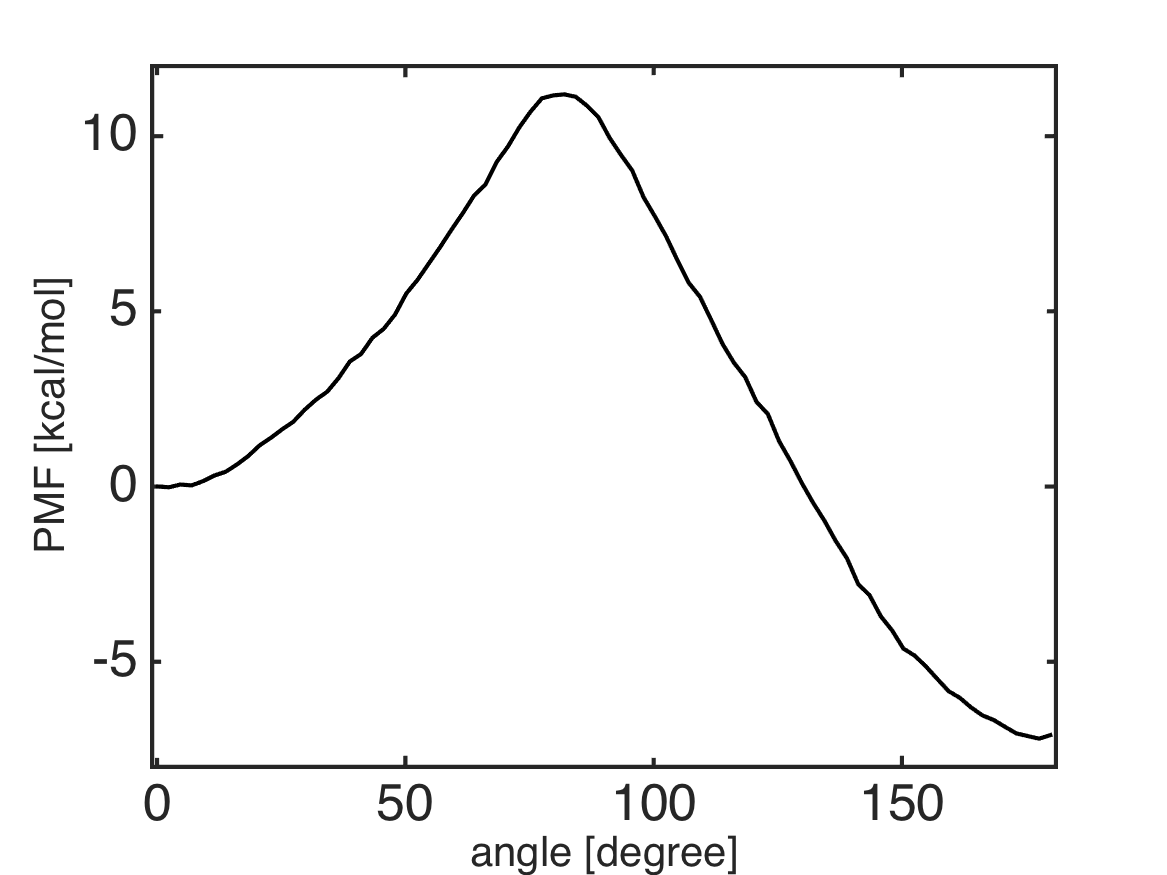# 1D Umbrella Sampling of Tri-Alanine and MBAR¶

Files for this example can be downloaded from here. This example is located in `mdtoolbox_example/umbrella_alat/mbar/`.

```% this routine calculates the Potential of Mean Force (PMF) from
% umbrella sampling data by using MBAR

%% constants
C = getconstants();
KBT = C.KB*300; % KB is the Boltzmann constant in kcal/(mol K)

%% define umbrella window centers
umbrella_center = 0:3:180;
K = numel(umbrella_center);

%% define function handles of bias energy for umbrella windows
for k = 1:K
spring_constant = 200 * (pi/180)^2; % conversion of the unit from kcal/mol/rad^2 to kcal/mol/deg^2
fhandle_k{k} = @(x) (spring_constant/KBT)*(minimum_image(x, umbrella_center(k))).^2;
end

data_k = {};
for k = 1:K
filename = sprintf('../3_prod/run_%d.dat', umbrella_center(k));
data_k{k} = x(:, 2);
end

%% evaluate u_kl: reduced bias-factor or potential energy of umbrella simulation data k evaluated by umbrella l
for k = 1:K
for l = 1:K
u_kl{k, l} = fhandle_k{l}(data_k{k});
end
end

%% MBAR
% calculate free energies of umbrella systems
f_k = mbar(u_kl);

%% PMF
M = 80; % number of bins
edge = linspace(-1, 181, M+1);
bin_center = 0.5 * (edge(2:end) + edge(1:(end-1)));
for k = 1:K
bin_k{k} = assign1dbin(data_k{k}, edge);
end
pmf = mbarpmf(u_kl, bin_k, f_k);
pmf = KBT*pmf;
pmf = pmf - pmf(1);

%% plot the PMF
hold off
plot(bin_center, pmf, 'k-');
formatplot
xlabel('angle [degree]', 'fontsize', 20);
ylabel('PMF [kcal/mol]', 'fontsize', 20);
axis([-1 181 -8 12]);
exportas('analyze')
hold off

%% save results
save analyze.mat;
```
```function dx = minimum_image(center, x)
dx = x - center;
dx = dx - round(dx./360)*360;
```